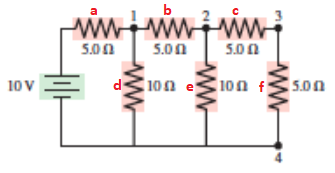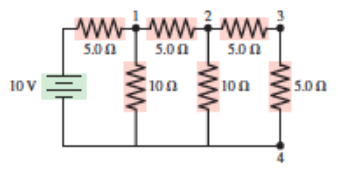# Problem: In the circuit at right, what is the potential at each of the noted points?

###### FREE Expert Solution

Equivalent resistance for resistors in parallel:

$\overline{)\frac{\mathbf{1}}{{\mathbf{R}}_{\mathbf{eq}}}{\mathbf{=}}\frac{\mathbf{1}}{{\mathbf{R}}_{\mathbf{1}}}{\mathbf{+}}\frac{\mathbf{1}}{{\mathbf{R}}_{\mathbf{2}}}{\mathbf{+}}{\mathbf{.}}{\mathbf{.}}{\mathbf{.}}{\mathbf{+}}\frac{\mathbf{1}}{{\mathbf{R}}_{\mathbf{n}}}}$

Equivalent resistance for resistors in series:

$\overline{){{\mathbf{R}}}_{{\mathbf{eq}}}{\mathbf{=}}{{\mathbf{R}}}_{{\mathbf{1}}}{\mathbf{+}}{{\mathbf{R}}}_{{\mathbf{2}}}{\mathbf{+}}{\mathbf{.}}{\mathbf{.}}{\mathbf{.}}{\mathbf{+}}{{\mathbf{R}}}_{{\mathbf{n}}}}$

Current:

$\overline{){\mathbf{i}}{\mathbf{=}}\frac{\mathbf{V}}{\mathbf{R}}}$Resistors C and Fare in series:

Rcf = Rc + Rf = 5.0 + 5.0 = 10Ω

Resistors CF and E are in parallel:

Rcfe = (1/Rcf + 1/Re)-1 = (1/10 + 1/10)-1 = 5.0Ω

Resistors CFE and B are in series:

Rcfeb = Rcfe + Rb = 5.0 + 5.0 = 10Ω

97% (157 ratings)###### Problem Details

In the circuit at right, what is the potential at each of the noted points?